# Detecting reactions from MD trajectories: ChemTraYzer2¶

## Introduction¶

Molecular dynamics (MD) simulations are a common way to simulate the time evolution of larger chemical systems. Because MD is often based on classical mechanics, systems can comprise thousands of interacting atoms, making it possible to study a variety of physical and chemical phenomena.

When simulating a reactive system – where chemical bonds between atoms can change over time – it is not uncommon to observe a very large number of reactions. For large or complex simulations, the many reactive events make it challenging to derive meaningful information from the trajectory. ChemTraYzer2 (CT2) is a tool designed to help with this problem. CT2 reads each frame of a reactive MD trajectory, keeps track of all reactions that occur, and summarizes all of this information into several useful quantities: a list of unique reactions, reaction rate constants for these reactions, the net fluxes of each species encountered in the simulation, and other kinetic and population measures.

## Summary of this tutorial¶

With this tutorial, we show how to …

• … set up a typical reactive gas simulation with a lot of reactions
• … run it with the molecular dynamics engine ReaxFF
• … set up ChemTraYzer 2 in the AMS GUI
• … find the reactions of the simulation
• … get rate constants for those reactions
• … visualize single reactive events in detail

We will start by setting up a common reactive molecular dynamics (MD) simulation. In our case we will simulate the combustion of hydrogen in a stoichiometric hydrogen/oxygen mixture, following this balanced reaction equation:

$\mathrm{2 H_2 + O_2 = 2 H_2O}$

Of course, hydrogen and oxygen will not react directly in this way, but rather via some intermediates like $$\mathrm{H}$$, $$\mathrm{OH}$$, and $$\mathrm{HO_2}$$, for example, by these elementary steps:

$\begin{split}\mathrm{H_2 + O_2} & \rightarrow \mathrm{HO_2 + H} \\ \mathrm{HO_2} & \rightarrow \mathrm{O + OH} \\ \mathrm{OH + H} & \rightarrow \mathrm{H_2O}\end{split}$

For this tutorial, we will choose an extreme temperature and density, because then the combustion reactions will happen faster and we can observe them within the time for this tutorial. When burning hydrogen, some types of reactions are expected to happen, like oxidation of hydrogen and decomposition reactions of oxidated products (as shown above). High temperatures usually favor decomposition pathways, but since we will have a lot of small molecules, we can expect to observe both oxidations and decompositions. Apart from that, the number of hydrogen/oxygen atom combinations in the molecules is quite limited and the resulting reaction network will not explode in size, even though we will simulate in those wild conditions.

## Step 1: set up a reactive hydrogen / oxygen combustion simulation¶

Start AMSinput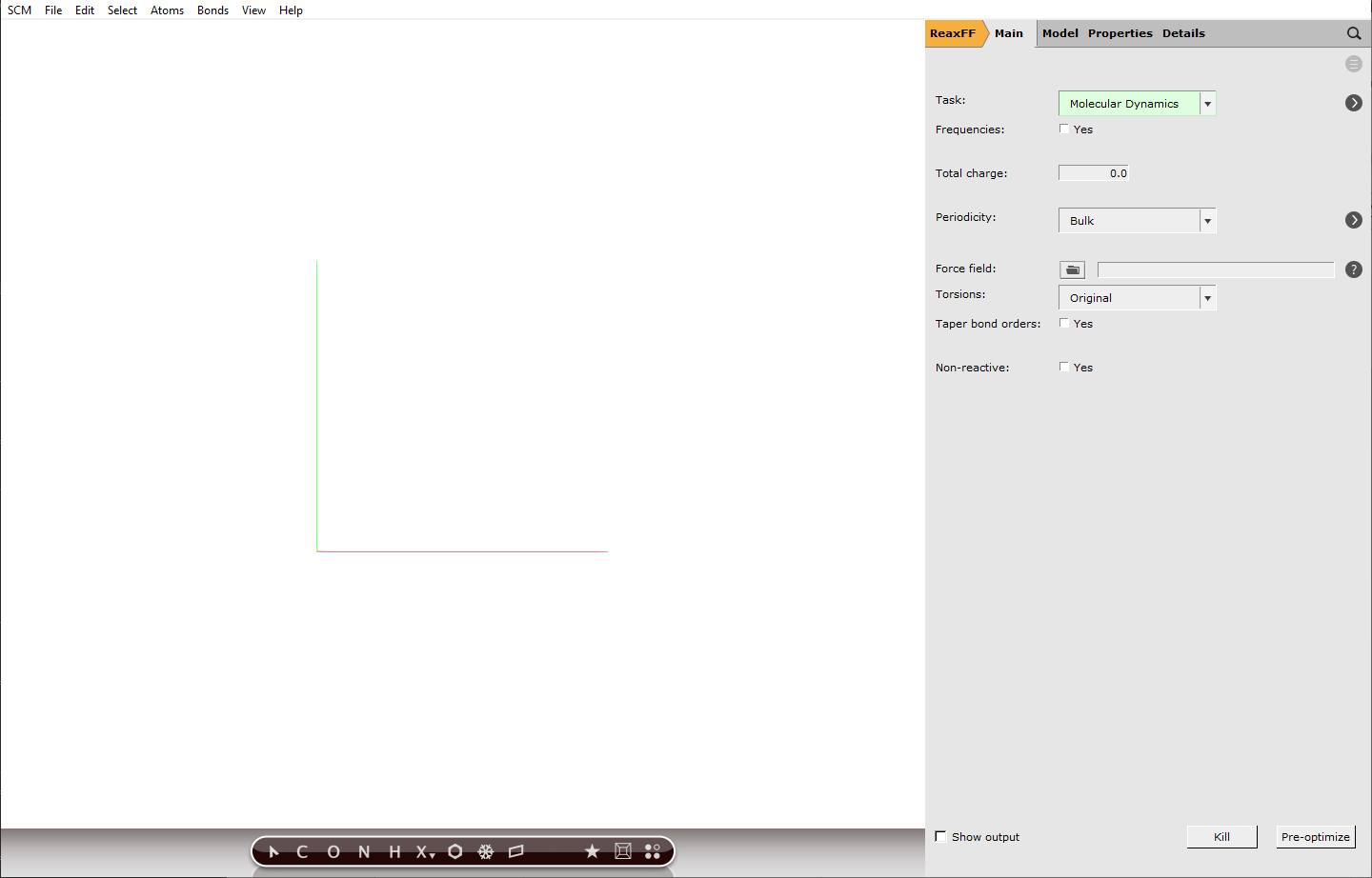### Building a periodic box of gas¶

To build our periodic box that contains our gas mixture, we will use the Builder tool of AMS. The Builder helps us to set up a initial stoichiometric composition and distribute our molecules in the box. We will simulate in quite a small box, so that the runtime of our simulation will be short.

Note

By selecting ReaxFF, molecular dynamics of a periodic box is chosen as a default setting.

Edit → Builder
Enter 15 as the length of the first lattice vector, the other vectors will adapt automatically
Enter “O2” in the field next to copies of, then select O2 (ADF) from the dropdown
Change the number of oxygen molecules from 100 to 20
Like before, enter “H2” and select H2: Hydrogen (ADF) from the dropdown
Change the hydrogen amount to 40
Click Generate Molecules
Close the Builder### Choosing simulation conditions¶

With the Builder tool, we already set the density of our system to $$60 / 15^3 \mathrm{molecules/{A^3}}$$ which translates to $$29.5 \mathrm{kmol/{m^3}}$$. So together with a very high temperature, we will create supercritical conditions, to make the combustion reactions happen faster. This will definitely not be a realistic setup, but it helps to see some reactions within a short calculation time.

Furthermore, we will use the default integration time step of 0.25 fs. With extreme conditions like in our case, the chemistry will be fast and usually smaller integration time steps are recommended to capture the molecular vibrations correctly. However, this would also increase the computation time, which is why we stick to the default value.

Model → MD or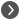next to Task: Molecular Dynamics
Set Number of steps to 200000
Set Sample frequency to 10
Set Initial temperature to 3500 K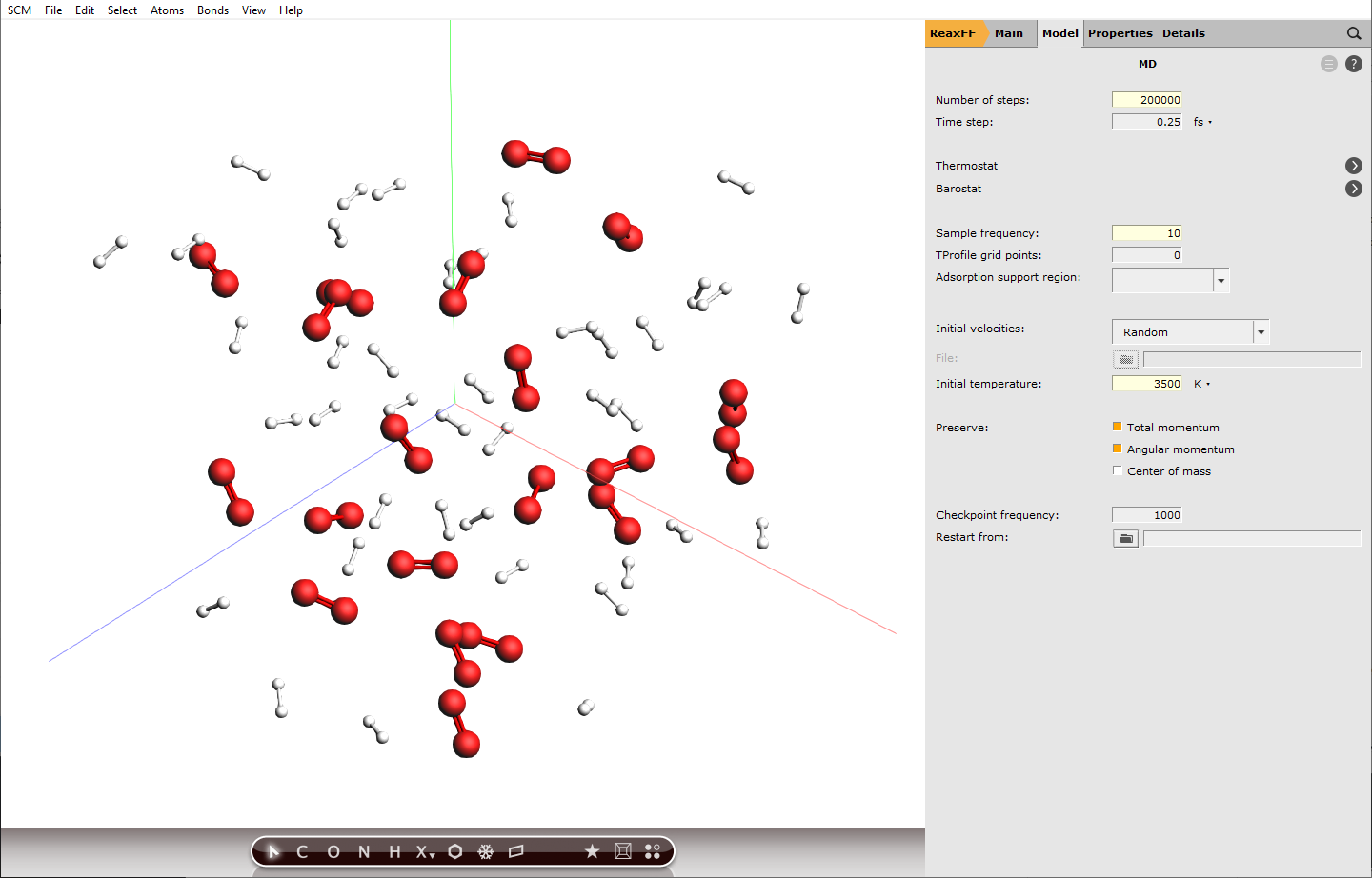Setting the sample frequency to 10 means every 10th calculated step is written to disk and can be analyzed later, this translates to a time difference of 2.5 fs between frames. Decreasing this value will increase the accuracy of the analysis later, but also the disk usage.

Note

200000 calculation steps at a step size of 0.25 fs translate to 50 ps of simulation.

### Setting up a thermostat¶

ChemTraYzer 2 can calculate rate constants for all observed reactions, by evaluating the number of events and the concentration of reactants for each reaction. The computation assumes constant temperature (an NVT ensemble), which is why we need to set up a thermostat that balances out temperature changes once reactions start to happen.

Model → Thermostat ornext to Thermostat
Add a thermostat by clickingand select NHC from the dropdown next to Thermostat:
Set the same temperature as before, which was 3500 K
Set Damping constant to 10 fs## Step 2: Run the simulation with ReaxFF¶

Click Main
Click on the folder button next to Force field:
Choose CHON-2019.ff, which was designed for hydrocarbon combustion and fits our case

Note

The list of ReaxFF parameterizations that appears when you click the folder button, will always contain parameters for the elements that are currently in the box.

Tip

Before actually running the simulation, we can check our settings. Details → Run Script summarizes the calculation job that is fed to the AMS calculation engine. All settings which are not default values are listed here. If we forgot to set a crucial setting, the program will notify us here with a pop-up warning.

File → Run from the AMSinput menu
Save the job as hydrogen.ams

Running this calculation takes approximately 10 minutes. You can do the following steps while the job is running.

AMSjobs should come to the foreground, and your job should be visible at the top. On the right side you can see that the job is running (this is indicated by the gear-icon). When running, in the AMSjobs window the progress of your simulation is showing (from the logfile). When you click on the progress lines the logfile will open in its own window:

Click on the logfile lines in the AMSjobs window to open the logfile

As you can see in the logfile, the simulation is running. To see more details, we now will use AMSmovie. Note that you can do this while the simulation is still running!

Start AMSmovie: SCM → Movie in the logfile window
Press the Play button (the triangle pointing right at the left bottom of the AMSmovie window)

AMSmovie will show you the trajectory of your system. Note that it will automatically read new data as soon as it becomes available. It can also show graphs of the properties that ReaxFF calculates: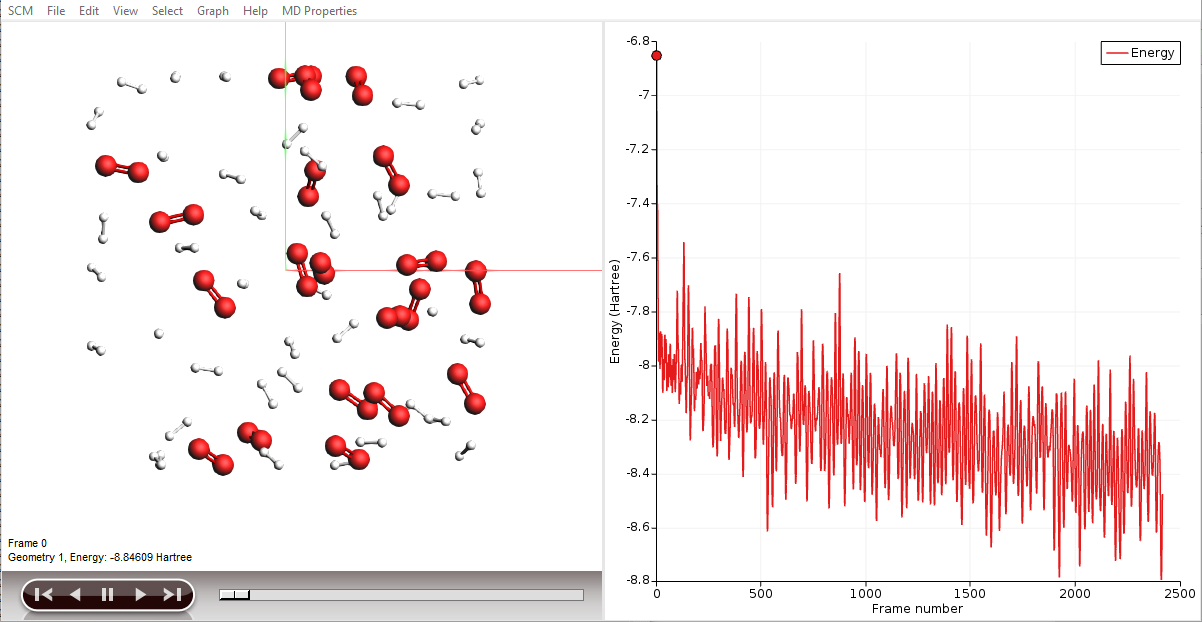In the AMSmovie window:
Use the MD Properties → Temperature command
Use the MD Properties → Potential Energy command
Use the MD Properties → Kinetic Energy commandNote

When reactions break up bonds, energy is released that was previously chemically bound. As a consequence, the kinetic energy of the system increases and the potential energy decreases. If we had not applied a thermostat, the temperature would rise together with the kinetic energy. The thermostat however slows down each atom from the system while it is heating up, which is why the temperature stays constant and the potential and total energy decrease.

Tip

You can drag the legend in the plotting area around with the mouse

You can go to a particular point in the simulation using the slider below the window showing your system, or you can click somewhere on one of the curves plotted. You can also use the arrow keys (left and right) to move through the simulation. Jump to the end of the movie to follow the progress.

## Step 3: Extract a list of reactions with ChemTraYzer 2¶

To start with this step, first wait for the calculation to finish. In the AMSlog window you can see the number of calculated steps, which eventually reaches 200000. Once that is done, a pop-up appears in the AMSinput window, asking to update the coordinates with the last frame of the simulation. Click yes and use the mouse buttons to look at the box. Some new species should have appeared, like $$\mathrm{OH}$$ and $$\mathrm{H_2O}$$. We didn’t put them in, so they were formed by reactions.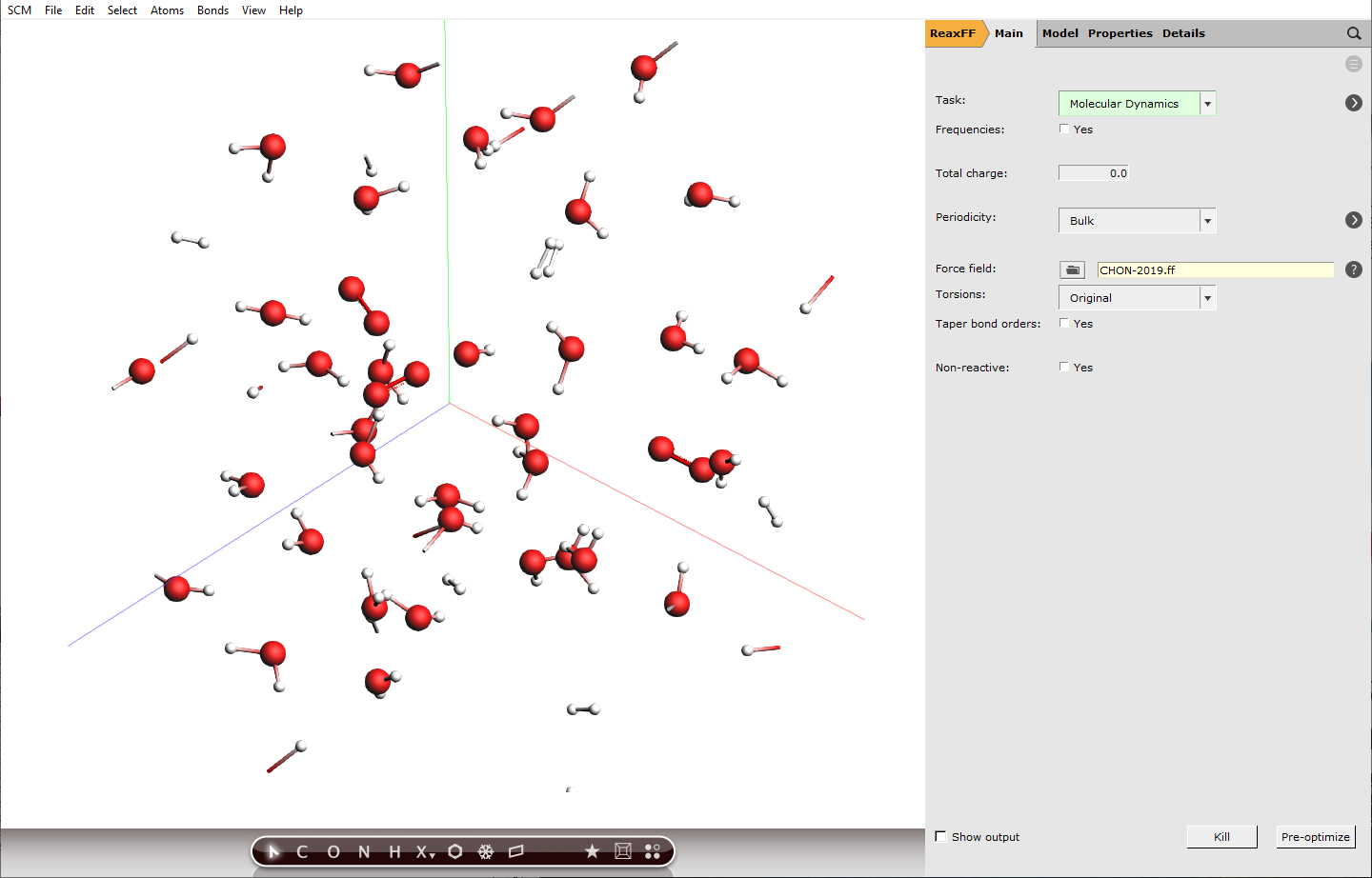Note

ChemTraYzer 2 can analyze any periodic gas calculation done with ReaxFF. Just open the RKF file in AMSmovie, and start ChemTraYzer 2 as explained below.

Let us see if we are able to find the types of reactions we expected in the beginning.

Switch to or open the AMSmovie window
MD Properties → Reaction Detection: ChemTraYzer 2

A new window opens, with settings for the trajectory analysis by ChemTraYzer 2. At first, we want to keep the standard settings as they are.Click Run ChemTraYzer 2
A progress window appears which shows that the simulation is being analyzed in the background
Once finished, click Show ResultsA new window opens with a list of the reactions found by ChemTraYzer 2. This window is split into two sections. The upper one lists all reactions by name, while the lower one lists each reaction’s individual occurrences ordered by time.

The reactions are reported in SMILES format and as molecular formulas, together with their reaction order. For each reaction, a rate constant is calculated, as well as an upper and lower bound to it. The first reaction you see in both lists should be some chain initiation step, e.g.:

$\mathrm{H_2 + O_2 \rightarrow H + HO_2}$

The last two columns contain the number of times a certain reaction was detected and its list of individual reaction event numbers. On the lower right side of each list, you can find a button to open it as a csv file (comma separated values), in case you want to process the data with another program or script.

The data can be sorted by every column.

Click the column header Number of events
Select Decreasing sort orderNow, the reactions that happened most frequently are on top of the list. In our setup, reactions of atomic hydrogen with molecular oxygen should be abundant, as estimated in the beginning of this tutorial. This is partly due to the fact that $$\mathrm{O_2}$$ can already act as a radical and is more stable than $$\mathrm{H_2}$$ under our conditions.

### Get reaction rate constants¶

For each reaction, ChemTraYzer 2 calculates a temperature-dependent rate constant based on the number of events and concentration of reactants during the simulation. You can see the calculated values in the upper list.

The units used here are cubic centimeters, mole, and second. So, for a bimolecular reaction the reported rate constant has a unit of $$\mathrm{cm^3*mol^{-1}*s^{-1}}$$, a termolecular rate constant has a unit of $$\mathrm{cm^6*mol^{-2}*s^{-1}}$$ and so on.

Let’s now compare two of the calculated rate constant to values from literature. For our temperature of 3500 K, there are no direct measurements, but we can still extrapolate a given Arrhenius equation to our temperature to get at least an idea of the right magnitude. For the reaction

$\mathrm{H_2 + O_2 \rightarrow H + HO_2}$

we find a value of $$4.2*10^{10}$$ from a 1986 review  and $$1.4*10^{11}$$ from a theoretical high-pressure-limit rate equation . All rate constant units mentioned here are $$\mathrm{cm^3*mol^{-1}*s^{-1}}$$, and the Arrhenius parameters are found via the NIST kinetics database. To find the respective value calculated by ChemTraYzer 2, do the following steps.

Click the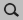search field from the upper list
Enter @Reaction Comp:O2 + H2 => to filter for reactions that consume $$\mathrm{O}_2$$ and $$\mathrm{H}_2$$
In column Reaction Composition, look for the entry O2 + H2 => H + HO2
Look for the Rate Constant of this reaction

In this example, we found a value of $$7*10^{11}$$, which is higher than the extrapolated literature values. Let’s check a second reaction

$\mathrm{H_2 + OH \rightarrow H_2O + H}$
Enter @Reaction Comp:H2 + HO => in thesearch field
Look for the rate constant of the entry H2 + HO => H + H2O

An extrapolated literature value for this rate constant is $$1.1*10^{13}$$ , whereas in this example, we have calculated $$4*10^{13}$$, which is again higher.

We can think of a few reasons, why our calculated values are off:

• We have hot and dense conditions, so the molecules have not enough time to always equilibrate in the beginning of the simulation and between reactions.
• The classical dynamics model ReaxFF cannot cover all quantum mechanical effects, and brings in some uncertainty.
• In this tutorial, the simulation size is small and the duration is short, so the rate constants are not representative.
• Our literature values were extrapolated.

However, the rate constant calculation by ChemTraYzer 2 comes with very little computational costs, and can be used as a first estimate and for comparison.

Note

As mentioned above, the simulation conditions are far away from an thermal equilibrium, and all the reactions are too fast (which we intended). Usually, a reactive mixture is first equilibrated for about a nanosecond before you want to allow reactions to happen. In this case, ChemTraYzer 2 can be used to verify that no reactions occurred in the equilibration phase, by using the Final frame setting (see Documentation).

Note

ChemTraYzer 2 can also compute rate constants for MD simulations that were accelerated by Collective Variable-Driven Hyperdynamics (CVHD) (see our CVHD tutorial or the CVHD documentation)

### Visualize reactive events¶

We used the upper list, now let’s make use of the single reaction events from the lower list. Each entry there corresponds to a change in the molecular composition that happened at some point during the simulation (an event). One could say that the upper list is a summary of the lower list.

Click on a reaction in the upper listWhen a reaction entry is selected, the lower list automatically shows all events of that reaction. The search bar below the events list then shows the search query that is used as a filter, e.g. (“@Index@3 45@”)

One of the reactions we expected to happen was $$\mathrm{HO_2 \rightarrow O + OH}$$. Let’s use the search function to find its first occurrence.

Press the ESC key to deselect the reaction
Click thesearch field from the lower list
Enter @Products:[OH]

Now, only reactions with a hydroxyl radical $$\mathrm{OH}$$ as a product are shown. Note here, that we have used the radical’s SMILES format for filtering (“[OH]”).

Click on the first entry of the lower list
Switch to the AMSmovie window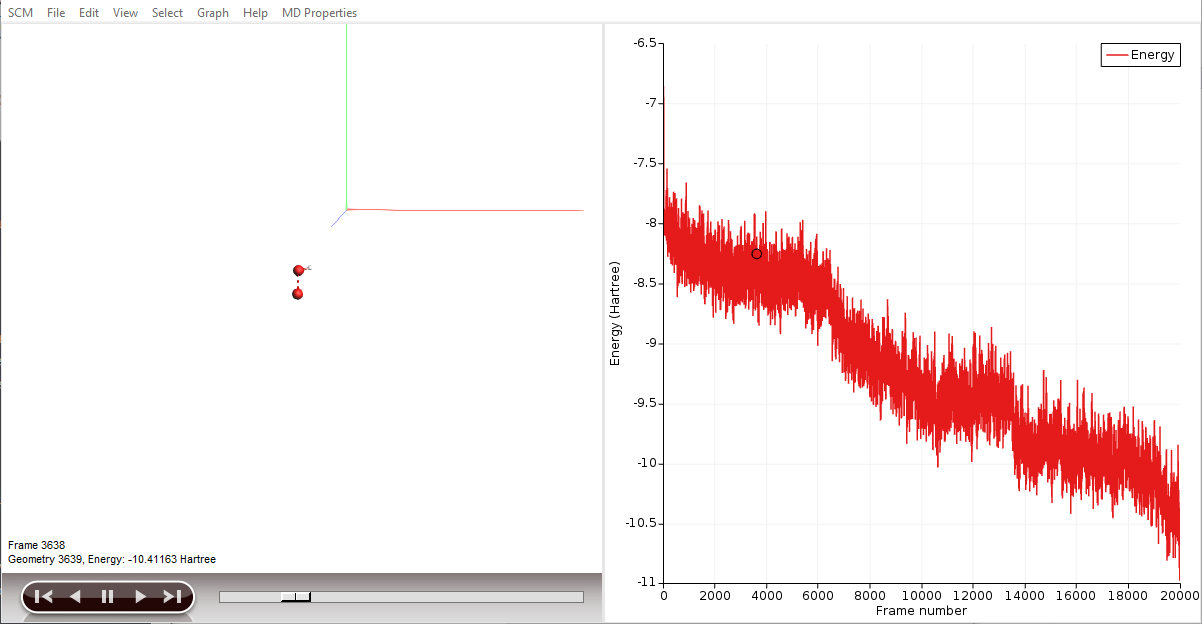By clicking an entry of the events list, AMSmovie automatically hides all atoms that are not involved in this particular event. Now, we can take a look in detail what led to the first production of $$\mathrm{OH}$$.

Zoom in on the visible atoms
use left and right keys to see the reaction taking place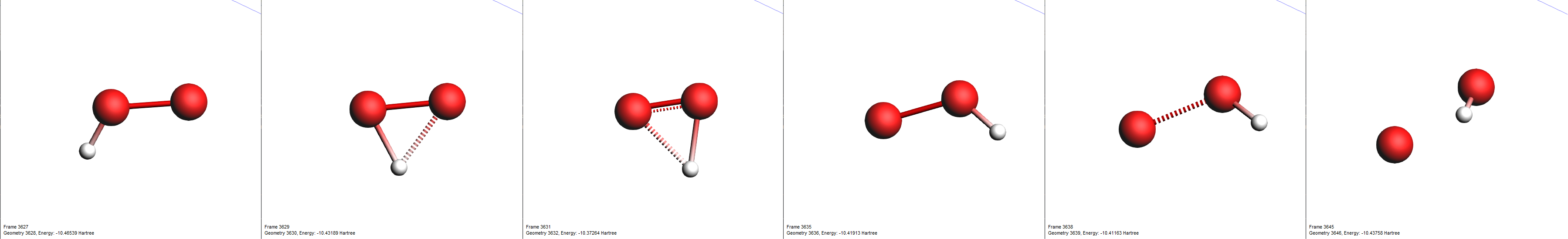Switch to the ChemTraYzer 2 results window
Press the ESC key to deselect any reactions and make all atoms reappear in AMSmovie
Press the ESC key again to clear thesearch field

### Using the “T_stable” setting¶

As mentioned in the introduction, the engine we used, ReaxFF, is a classical force field and therefore it does not include electrons or orbitals. Especially in our dense simulation, some unintuitive or unexpected molecules may appear, like $$\mathrm{HOHOH}$$ or $$\mathrm{OH_3}$$. These might be artifacts of an insufficient accuracy of the force field parameters or actual short-lived unstable intermediates.

In the latter case, we can increase the analysis setting T stable to a higher number. This setting determines how much time ChemTraYzer 2 gives the products to settle into a stable state, and register the reaction.

In this section of the tutorial, we will look for the unstable intermediate $$\mathrm{HOHOH}$$ and increase $$\mathrm{t_{stable}}$$ to see the effects on the reaction detection involving this intermediate.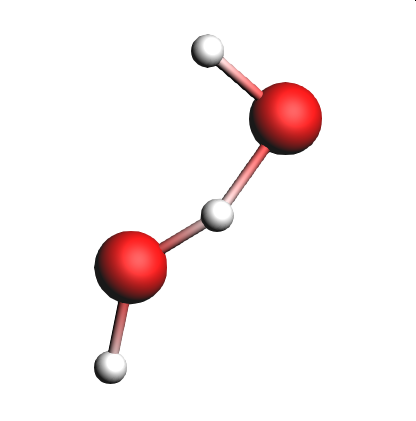Enter @Products:O[H]O in thesearch field of the lower list
Mind the number of found items

If we see $$\mathrm{HOHOH}$$ in one of lists, it means that the intermediate was present in the simulation for longer than the time specified by T stable.

Switch to the ChemTraYzer 2 settings window
Change T stable to 20
Click Run ChemTraYzer 2

Wait for the analysis to finish, and look look again for $$\mathrm{HOHOH}$$ in the reactions list.

Enter @Products:O[H]O in thesearch field of the lower list
Mind the number of found items

By increasing the time threshold of stable molecules, the number of unstable intermediates detected as reaction products should have decreased.